Knowledge Evolution in Neural Networks

Deep learning stands on two pillars: GPUs and large datasets. Thus, deep networks suffer when trained from scratch on small datasets. This phenomenon is known as overfitting. This paper  proposes knowledge evolution to reduces both overfitting and the burden for data collection. In the paper, knowledge evolution is supported by two intuitions: Dropout and ResNets. Yet, this article presents another third intuition — not mentioned in the paper.

Before delving into knowledge evolution (KE), let’s define the term overfitting. Overfitting happens when a neural network enters an inferior local minimum. At this minimum, the training loss decreases while the network performance over a validation/test split degenerates. Unfortunately, gradient descent cannot get the network out of an inferior local minimum. The next figure shows two local minima; both with zero gradients. If the network falls into the inferior local minimum (red), gradient descent will not get the network to the better local minimum (green).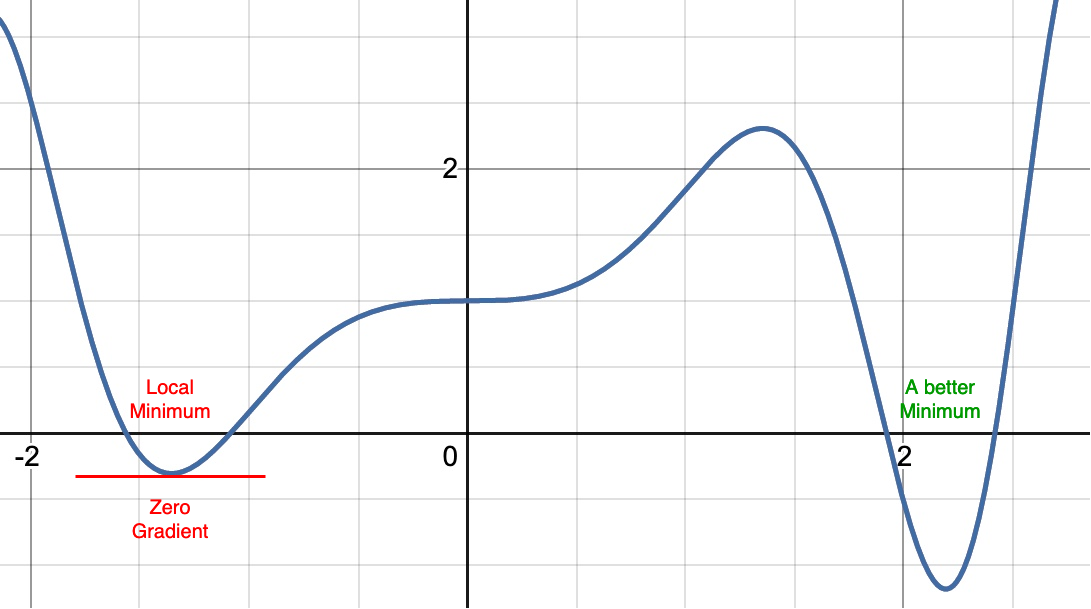The optimization manifold for a deep network has many local minima. With a small dataset, the deep network is likely to fall into an inferior local minimum. Within a local minimum, the gradient is zero. Accordingly, gradient descent cannot get the network out. Knowledge evolution (KE) splits the network into two parts and re-initializes one part randomly. Hopefully, this gets the network out of the inferior local minimum. Then, KE resumes training — using gradient descent — to evolve the knowledge inside the network.

To get out of an inferior local minimum, we can reinitialize the whole network. This will get the network out of the inferior local minimum, but it is back to square one! Instead, knowledge evolution (1) splits the network into two parts and (2) randomly re-initializes one of those parts. Accordingly, some knowledge (weights) is (are) retained in the non-reinitialized part. KE splits a given network into two parts: the fit and reset hypotheses. The next figure shows the fit-hypothesis in blue and the reset-hypothesis in gray.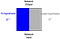KE splits a deep network into two parts: fit-hypothesis (blue) and reset-hypothesis (gray). KE re-initializes the reset-hypothesis when the network overfits, i.e., enters an inferior local minimum. After re-initialization, the network resumes training using gradient descent. Gradient descent evolves the knowledge inside the fit-hypothesis.

KE trains a network for multiple generations, each with e epochs. After each generation, KE re-initializes the reset-hypothesis, then trains the next generation as shown in the next figure.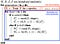After training a network for e epochs, KE reinitializes the reset-hypothesis. Then, KE trains the next generations iteratively.

This simple idea reduces overfitting and boosts performance on small datasets. The next figure presents a quantitative evaluation using two small datasets: Flower102 and CUB200. The dashed-lines denote the cross-entropy performance on the two datasets, while the marked-curves show the knowledge evolution’s performance across generations. The 100-th generation achieves absolute 21% and 5% improvement margins over the Flower-102 and CUB-200 baselines, respectively.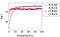Classification performance on Flower-102 (FLW) and CUB-200 (CUB) datasets trained on a randomly initialized ResNet18. The horizontal dashed lines denote a SOTA cross-entropy (CE) baseline . The marked curves show KE’s performance across generations.

Interestingly, KE achieves these significant improvement margins at a lower inference cost. While typical pruning methods compress a network after training, KE learns a slim (pruned) network during training. KE evolves the fit-hypothesis’s knowledge till it matches the whole network’s knowledge. The next figure illustrates how the fit-hypothesis’s knowledge evolves as the number of generations increases. The red crossed-curve denotes the performance of the whole dense network, while the blue dotted-curve denotes the fit-hypothesis’s performance. After three generations (g=3), the fit-hypothesis (one part) achieves comparable performance to the dense network (two parts). Yet, this performance is achieved at a lower inference cost.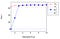Quantitative classification evaluation using CUB-200 on VGG11-bn. The x-axis denotes the number of generations. The fit-hypothesis (blue dotted curve) achieves an inferior performance at g = 1, but its performance increases as the number of generations increases.

The next table highlights both the absolute accuracy improvement (green) and relative computational reduction (blue) achieved by KE. KE achieves significant improvement margins — up to an absolute 21% while reducing inference cost by a relative 73.5%.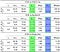Quantitative evaluation for KE using the number of both operations (G-Ops) and parameters (millions). Acc_g denotes the classification accuracy at the g-th generation. \triangle_ops denotes the relative reduction in the number of operations. \triangle_acc denotes the absolute accuracy improvement on top of the dense baseline N1.

While KE is proposed for small datasets, KE also supports large datasets (e.g., ImageNet). The next table evaluates KE on ImageNet using two architectures: ResNet34 and ResNet50. KE boosts the performance of both the dense network and the fit-hypothesis.

[S1] The paper is well-written and the authors released their code.

[S2] The paper presents a ton of experiments in both the manuscript and the paper appendix.

[W1] The paper focuses on convolutional neural networks. I wish the authors evaluated KE using graph convolution networks (GCNs). KE should have a big impact on GCNs because they are typically randomly initialized.

[W2] The paper achieves its best results on the Flower102 dataset. This is probably because Flower102 is both a small and a coarse-grained dataset. I wish there are more datasets with similar characteristics to confirm the efficiency of KE.

References

 Taha, A., Shrivastava, A., & Davis, L. S. Knowledge Evolution in Neural Networks. CVPR2021.

 Yun, S., Park, J., Lee, K., & Shin, J. Regularizing class-wise predictions via self-knowledge distillation. CVPR2020.

I write reviews on computer vision papers. Writing tips are welcomed.